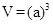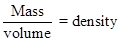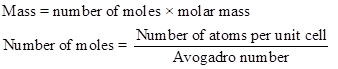Chapter 12.1, Problem 12.1CYU

Chapter
Section
Textbook Problem

(a) Determining an Atom Radius from Lattice Dimensions: Gold has a face-centered unit cell, and its density is 19.32 g/cm3. Calculate the radius of a gold atom. (b) The Structure of Solid Iron: Iron has a density of 7.8740 g/cm3, and the radius of an iron atom is 126 pm. Verify that solid iron has a body-centered cubic unit cell. (Be sure to note that the atoms in a body-centered cubic unit cell touch along the diagonal across the cell. They do not touch along the edges of the cell.) (Hint: The diagonal distance across the unit cell = edge × 3 .)

(a)

Interpretation Introduction

Interpretation:

The radius of the gold atom has to be calculated.

Concept introduction:

The volume of the atom is given below,The density of the unit cell is calculated as follows,Explanation

Therefore,

(b)

Interpretation Introduction

Interpretation:

Solid iron has a body-centered cubic unit cell of iron atom has to be verified.

Concept introduction:

The volume of the atom is given below,The density of the unit cell is calculated as follows,Still sussing out bartleby?

Check out a sample textbook solution.

See a sample solution

The Solution to Your Study Problems

Bartleby provides explanations to thousands of textbook problems written by our experts, many with advanced degrees!

Get Started